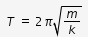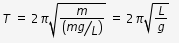# What is simple pendulum? find an expression for the time periods and frequency of a simple pendulum?

what is simple pendulum? find an expression for the time periods and frequency of a simple pendulum?

A simple pendulum is an idealized body consisting of a particle suspended by a light inextensible cord. When pulled to one side of its equilibrium position and released, the pendulum swings in a vertical plane under the influence of gravity. The motion is periodic and oscillatory.Let us consider the simple pendulam makes an angular displacement θ as shown in figure. At this position the forces are weight of the bob of pendulam and Tension acting on the string as shown in figure. Let us resolve the weight mg, the gravitational force into two perpendicular components as shown in figure. one component mgcosθ is in the direction of tension T, that equalize the tension which is giving necessary centripetal force for circular motion. The other perpendicular component mgsinθ is the restoring force acting on the bob to bring to its equilibrium position.
The restoring force Fx is written as, Fx = -mgsinθ (-ve sign indicates, the displacement is in opposite direction to force )
for small angular displacement sinθ ≈ θ, hence Fx = -mgθ = -mg (x/L) = -(mg/L)x …(1)
here we have assumed the displacement x is nearly equal to the arc length Lθ .
Above eqn(1) indicates the restoring force is directly proportional to displacement, hence the oscillatory motion is simple harmonic.
we know that for simple harmonic motion, F = -kx, where k is the force constant and period T of oscillation is given by,we can see that the bracketed term of the right hand side of equation(1) is the force constant for the simple harmonic motion of pendulam.
hence we can write for the period T of simple pendulam as,frequency =